# Excel FIND and SEARCH functions with formula examples

The tutorial explains the syntax of the Excel FIND and SEARCH functions and provides formula examples of advanced non-trivial uses.

In the last article, we covered the basics of the Excel Find and Replace dialog. In many situations, however, you may want Excel to find and extract data from other cells automatically based on your criteria. So, let's have a closer look at what the Excel search functions have to offer.

## Excel FIND function

The FIND function in Excel is used to return the position of a specific character or substring within a text string.

The syntax of the Excel Find function is as follows:

FIND(find_text, within_text, [start_num])

The first 2 arguments are required, the last one is optional.

• Find_text - the character or substring you want to find.
• Within_text - the text string to be searched within. Usually it's supplied as a cell reference, but you can also type the string directly in the formula.
• Start_num - an optional argument that specifies from which character the search shall begin. If omitted, the search starts from the 1st character of the within_text string.

If the FIND function does not find the find_text character(s), a #VALUE! error is returned.

For example, the formula `=FIND("d", "find")` returns 4 because "d" is the 4th letter in the word "find". The formula `=FIND("a", "find")` returns an error because there is no "a" in "find".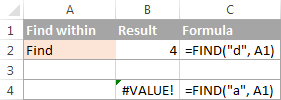### Excel FIND function - things to remember!

To correctly use a FIND formula in Excel, keep in mind the following simple facts:

1. The FIND function is case sensitive. If you are looking for a case-insensitive match, use the SEARCH function.
2. The FIND function in Excel does not allow using wildcard characters.
3. If the find_text argument contains several characters, the FIND function returns the position of the first character. For example, the formula FIND("ap","happy") returns 2 because "a" in the 2nd letter in the word "happy".
4. If within_text contains several occurrences of find_text, the first occurrence is returned. For example, FIND("l", "hello") returns 3, which is the position of the first "l" character in the word "hello".
5. If find_text is an empty string "", the Excel FIND formula returns the first character in the search string.
6. The Excel FIND function returns the #VALUE! error if any of the following occurs:
• Find_text does not exist in within_text.
• Start_num contains more characters than within_text.
• Start_num is 0 (zero) or a negative number.

## Excel SEARCH function

The SEARCH function in Excel is very similar to FIND in that it also returns the location of a substring in a text string. Is syntax and arguments are akin to those of FIND:

SEARCH(find_text, within_text, [start_num])

Unlike FIND, the SEARCH function is case-insensitive and it allows using the wildcard characters, as demonstrated in the following example.

And here's a couple of basic Excel SEARCH formulas:

`=SEARCH("market", "supermarket")` returns 6 because the substring "market" begins at the 6th character of the word "supermarket".

`=SEARCH("e", "Excel")` returns 1 because "e" is the first character in the word "Excel", ignoring the case.

Like FIND, Excel's SEARCH function returns the #VALUE! error if:

• The start_num argument is greater than the length of within_text.
• Start_num is equal to or less than zero.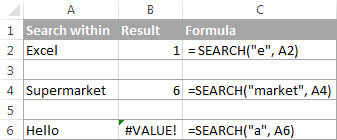Further on in this tutorial, you will find a few more meaningful formula examples that demonstrate how to use SEARCH function in Excel worksheets.

## Excel FIND vs. Excel SEARCH

As already mentioned, the FIND and SEARCH functions in Excel are very much alike in terms of syntax and uses. However, they do have a couple of differences.

#### 1. Case-sensitive FIND vs. case-insensitive SEARCH

The most essential difference between the Excel SEARCH and FIND functions is that SEARCH is case-insensitive, while FIND is case-sensitive.

For example, SEARCH("e", "Excel") returns 1 because it ignores the case of "E", while FIND("e", "Excel") returns 4 because it minds the case.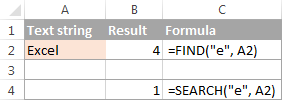#### 2. Search with wildcard characters

Unlike FIND, the Excel SEARCH function accepts wildcard characters in the find_text argument:

• A question mark (?) matches one character, and
• An asterisk (*) matches any series of characters.

To see how it works on real data, consider the following example: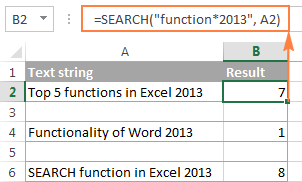As you see in the screenshot above, the formula SEARCH("function*2013", A2) returns the position of the first character ("f") in the substring if the text string referred to in the within_text argument contains both "function" and "2013", no matter how many other characters there are in between.

Tip. To find an actual question mark (?) or asterisk (*), type a tilde (~) before the corresponding character.

## Excel FIND and SEARCH formula examples

In practice, the Excel FIND and SEARCH functions are rarely used on their own. Typically, you would utilize them in combination with other functions such as MID, LEFT or RIGHT, and the following formula examples demonstrate some real-life uses.

### Example 1. Find a string preceding or following a given character

This example shows how you can find and extract all characters in a text string to the left or to the right of a specific character. To make things easier to understand, consider the following example.

Supposing you have a column of names (column A) and you want to pull the First name and Last name into separate columns.

To get the first name, you can use FIND (or SEARCH) in conjunction with the LEFT function:

`=LEFT(A2, FIND(" ", A2)-1)`

or

`=LEFT(A2, SEARCH(" ", A2)-1)`

As you probably know, the Excel LEFT function returns the specified number of left-most characters in a string. And you use the FIND function to determine the position of a space (" ") to let the LEFT function know how many characters to extract. At that, you subtract 1 from the space's position because you don't want the returned value to include the space.

To extract the last name, use the combination of the RIGHT, FIND / SEARCH and LEN functions. The LEN function is needed to get the total number of characters in the string, from which you subtract the position of the space:

`=RIGHT(A2,LEN(A2)-FIND(" ",A2))`

or

`=RIGHT(A2,LEN(A2)-SEARCH(" ",A2))`

The following screenshot demonstrates the result: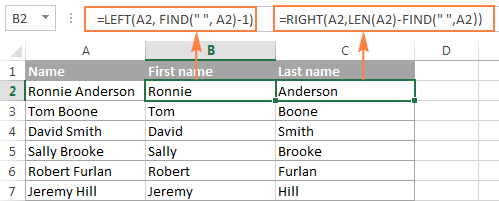For more complex scenarios, such as extracting a middle name or splitting names with suffixes, please see How to split cells in Excel using formulas.

### Example 2. Find Nth occurrence of a given character in a text string

Supposing you have some text strings in column A, say a list of SKUs, and you want to find the position of the 2nd dash in a string. The following formula works a treat:

`=FIND("-", A2, FIND("-",A2)+1)`

The first two arguments are easy to interpret: locate a dash ("-") in cell A2. In the third argument (start_num), you embed another FIND function that tells Excel to start searching beginning with the character that comes right after the first occurrence of dash (FIND("-",A2)+1).

To return the position of the 3rd occurrence, you embed the above formula in the start_num argument of another FIND function and add 2 to the returned value:

`=FIND("-",A2, FIND("-", A2, FIND("-",A2)+1) +2)`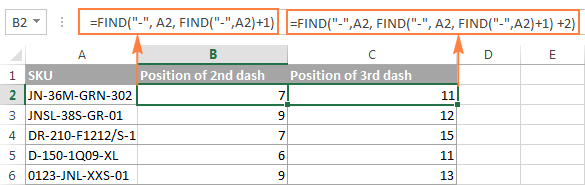Another and probably a simpler way of finding the Nth occurrence of a given character is using the Excel FIND function in combination with CHAR and SUBSTITUTE:

`=FIND(CHAR(1),SUBSTITUTE(A2,"-",CHAR(1),3))`

Where "-" is the character in question and "3" is the Nth occurrence you want to find.

In the above formula, the SUBSTITUTE function replaces the 3rd occurrence of dash ("-") with CHAR(1), which is the unprintable "Start of Heading" character in the ASCII system. Instead of CHAR(1) you can use any other unprintable character from 1 to 31. And then, the FIND function returns the position of that character in the text string. So, the general formula is as follows:

FIND(CHAR(1),SUBSTITUTE(cell,character,CHAR(1),Nth occurrence))

At first sight, it may seem that the above formulas have little practical value, but the next example will show how useful they are in solving real tasks.

Note. Please remember that the Excel FIND function is case-sensitive. In our example, this makes no difference, but if you are working with letters and you want a case-insensitive match, use the SEARCH function instead of FIND.

### Example 3. Extract N characters following a certain character

To locate a substring of a given length within any text string, use Excel FIND or Excel SEARCH in combination with the MID function. The following example demonstrates how you can use such formulas in practice.

In our list of SKUs, supposing you want to find the first 3 characters following the first dash and pull them in another column.

If the group of characters preceding the first dash always contains the same number of items (e.g. 2 chars) this would be a trivial task. You could use the MID function to return 3 characters from a string, starting at position 4 (skipping the first 2 characters and a dash):

`=MID(A2, 4, 3)`

Translated into English, the formula says: "Look in cell A2, begin extracting from character 4, and return 3 characters".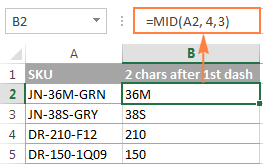However, in real-life worksheets, the substring you need to extract could start anywhere within the text string. In our example, you may not know how many characters precede the first dash. To cope with this challenge, use the FIND function to determine the starting point of the substring that you want to retrieve.

The FIND formula to return the position of the 1st dash is as follows:

`=FIND("-",A2)`

Because you want to start with the character that follows the dash, add 1 to the returned value and embed the above function in the second argument (start_num) of the MID function:

`=MID(A2, FIND("-",A2)+1, 3)`

In this scenario, the Excel SEARCH function works equally well:

`=MID(A2, SEARCH("-",A2)+1, 3)`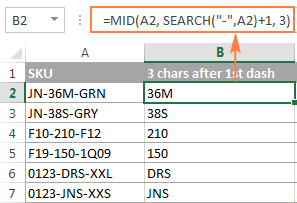It's great, but what if the group of chars following the first dash contains a different number of characters? Hmm... this might be a problem: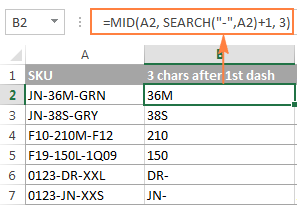As you see in the above screenshot, the formula works perfectly for rows 1 and 2. In rows 4 and 5, the second group contains 4 characters, but only the first 3 chars are returned. In rows 6 and 7, there are only 2 characters in the second group, and therefore our Excel Search formula returns a dash following them.

If you wanted to return all chars between the 1st and 2nd occurrences of a certain character (dash in this example), how would you proceed? Here is the answer:

`=MID(A2, FIND("-",A2)+1, FIND("-", A2, FIND("-",A2)+1) - FIND("-",A2)-1)`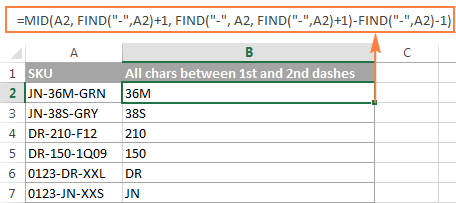For better understanding of this MID formula, let's examine its arguments one by one:

• 1st argument (text). It's the text string containing the characters you want to extract, cell A2 in this example.
• 2nd argument (start_position). Specifies the position of the first character you want to extract. You use the FIND function to locate the first dash in the string and add 1 to that value because you want to start with the character that follows the dash: FIND("-",A2)+1.
• 3rd argument (num_chars). Specifies the number of characters you want to return. In our formula, this is the trickiest part. You use two FIND (or SEARCH) functions, one determines the position of the first dash: FIND("-",A2). And the other returns the position of the second dash: FIND("-", A2, FIND("-",A2)+1). Then you subtract the former from the latter, and then subtract 1 because you don't want to include either dash. As the result, you will get the number of characters between the 1st and 2nd dashes, which is exactly what we are looking for. So, you feed that value to the num_chars argument of the MID function.

In a similar fashion, you can return 3 characters after the 2nd dash:

`=MID(A2, FIND("-",A2, FIND("-", A2, FIND("-",A2)+1) +2), 3)`

Or, extract all the characters between the 2nd and 3rd dashes:

`=MID(A2, FIND("-", A2, FIND("-",A2)+1)+1, FIND("-",A2, FIND("-", A2, FIND("-",A2)+1) +2) - FIND("-", A2, FIND("-",A2)+1)-1)`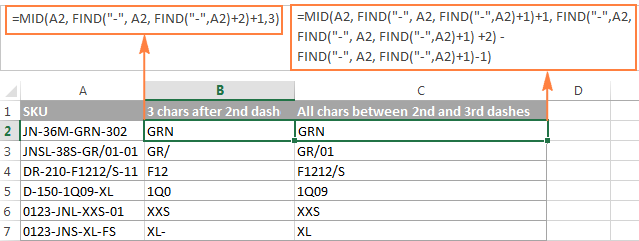### Example 4. Find text between parentheses

Supposing you have some long text string in column A and you want to find and extract only the text enclosed in (parentheses).

To do this, you would need the MID function to return the desired number of characters from a string, and either Excel FIND or SEARCH function to determine where to start and how many characters to extract.

`=MID(A2,SEARCH("(",A2)+1, SEARCH(")",A2)-SEARCH("(",A2)-1)`

The logic of this formula is similar to the ones we discussed in the previous example. And again, the most complex part is the last argument that tells the formula how many characters to return. That pretty long expression in the num_chars argument does the following:

• First, you find the position of the closing parenthesis: `SEARCH(")",A2)`
• After that you locate the position of the opening parenthesis: `SEARCH("(",A2)`
• And then, you calculate the difference between the positions of the closing and opening parentheses and subtract 1 from that number, because you don't want either parenthesis in the result: `SEARCH(")",A2)-SEARCH("(",A2))-1`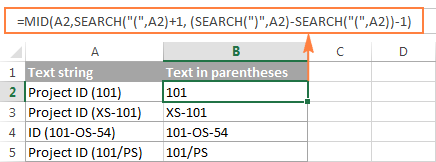Naturally, nothing prevents you from using the Excel FIND function instead of SEARCH, because case-sensitivity or case-insensitivity makes no difference in this example.

Hopefully, this tutorial has shed some light on how to use SEARCH and FIND functions in Excel. In the next tutorial, we are going to closely examine the REPLACE function, so please stay tuned. Thank you for reading!